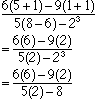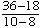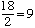Mildred Saulsbery elemantary alg. Student Find the value of the expression. ```6(5+1) - 9(1+1) _____________ 5(8 - 6) -23 ``` Hi Mildred, When evaluating a mathematical expression evaluation should take place in the following order. Operations that are inside Parentheses Powers (Exponents) Division and Multiplication Addition and Subtraction (some people remember this as PEDMAS.) With your expression performing the operations in this order givesAt this point you might wonder what to do next. The rules above suggest that you divide, but you can't because you don't have a numeric value for the numerator or denominator. Multiplication givesAgain you can't perform the division without evaluating the numerator and denominator. This evaluation givesand thus ```6(5+1) - 9(1+1) _____________ 5(8 - 6) -23 ``` =9 Cheers, Harley Go to Math Central